# Travelling Salesman Problem using Branch and Bound

Given a set of cities and distance between every pair of cities, the problem is to find the shortest possible route that visits every city exactly once and returns to the starting point.

For example, consider below graph. A TSP tour in the graph is A -> B -> C -> D -> B -> A. The cost of the tour is 10 + 25 + 40 + 25 + 10 = 100.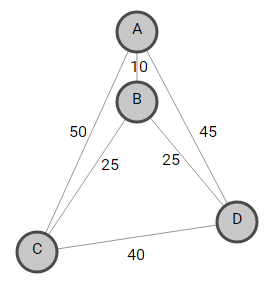In this post, Travelling Salesman Problem using Branch and Bound is discussed.

The term Branch and Bound refers to all state space search methods in which all the children of E-node are generated before any other live node can become the E-node. E-node is the node, which is being expended. State space tree can be expended in any method i.e. BFS or DFS. Both start with the root node and generate other nodes. A node which has been generated and all of whose children are not yet been expanded is called live-node. A node is called dead node, which has been generated, but it cannot be expanded further. In this method we expand the node, which is most promising, means the node which promises that expanding or choosing it will give us the optimal solution. So we prepare the tree starting form root then we expand it.

We have a cost Matrix defined by

C(i, j) = W(i, j), if there is a direct path from Ci to Cj
= INFINTY, if there is no direct path from Ci to Cj

For example, consider below cost matrix M,Here,
C(0, 2) = 30
C(4, 0) = 16
C(1, 1) = INFINITY

Below is state space tree for above TSP problem, which shows optimal solution marked in green.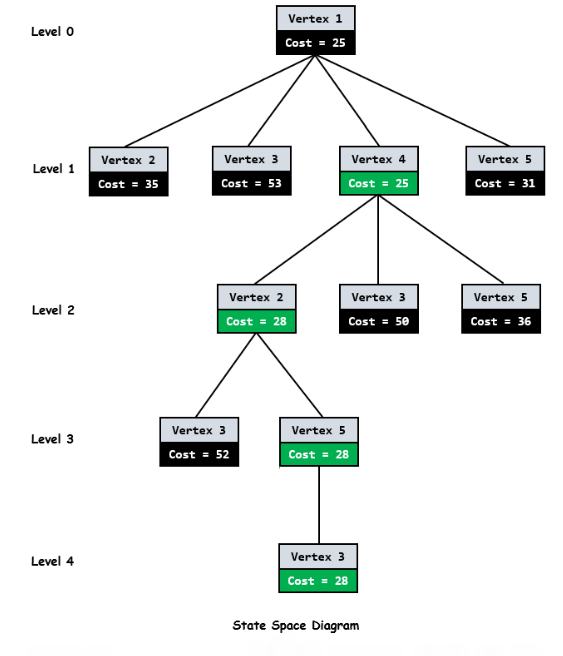As we can see from above diagram, every node has a cost associated to it. When we we go from city i to city j, cost of a node j will be sum of cost of parent node i, cost of the edge (i, j) and lower bound of the path starting at node j.

As root node is the first node to be expanded, it doesn’t have any parent. So cost will be only lower bound of the path starting at root.

Now how do we calculate lower bound of the path starting at any node?

In general, to get the lower bound of the path starting from the node, we reduce each row and column in such a way that there must be at least one zero in each row and Column. For doing this, we need to reduce the minimum value from each element in each row and column.

Let’s start from root node.

We reduce the minimum value in each row from each element in that row. Minimum in each Row of cost matrix M is marked by blue [10 2 2 3 4] below.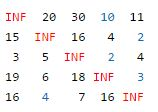After reducing the row, we get below reduced matrix.We then reduce the minimum value in each column from each element in that column. Minimum in each Column is marked by blue [1 0 3 0 0]. After reducing the column, we get below reduced matrix. This matrix will be further processed by child nodes of root node to calculate their lower bound.The total expected cost at the root node is the sum of all reductions.

Cost = [10 2 2 3 4] + [1 0 3 0 0] = 25

Since we have discovered the root node C0, the next node to be expanded can be any node from C1, C2, C3, C4. Whichever node has minimum cost, that node will be expanded further. So we have to find out the expanding cost of each node.

The parent node (C0) has below reduced matrix –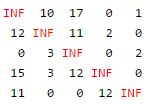Lets consider edge from 0 -> 1.

1. As we are adding edge (0, 1) to our search space, we set outgoing edges for city 0 to infinity and all incoming edges to city 1 to infinity. We also set (1, 0) to infinity.

So in reduced matrix of parent node, we change all the elements in row 0 and column 1 and at index (1, 0) to INFINITY (marked in red).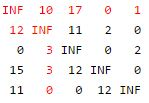The resulting cost matrix is: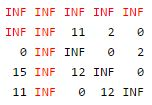2. We try to calculate lower bound of the path starting at node 1 using above resulting cost matrix. The lower bound is 0 as matrix is already in reduced form. i.e. all rows and all columns have zero value.

Therefore for node 1, cost will be
Cost = cost of node 0 +
cost of the edge(0, 1) +
lower bound of the path starting at node 1
= 25 + 10 + 0 = 35

Lets consider edge from 0 -> 2

1. Change all the elements in row 0 and column 2 and at index (2, 0) to INFINITY (marked in red).The resulting cost matrix is: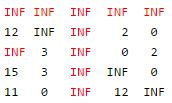2. Now calculate lower bound of the path starting at node 2 using the approach discussed earlier. Resultant matrix will be –Therefore for node 2, cost will be

Cost = cost of node 0 +
cost of the edge(0, 2) +
lower bound of the path starting at node 2
= 25 + 17 + 11 = 53

Lets consider edge from 0 -> 3.

1. Change all the elements in row 0 and column 3 and at index (3, 0) to INFINITY (marked in red).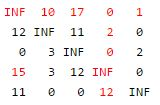The resulting cost matrix is: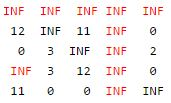2. Now calculate lower bound of the path starting at node 3 using the approach discussed earlier. Lower bound of the path starting at node 3 is 0 as it is already in reduced form. i.e. all rows and all columns have zero value.

Therefore for node 3, cost will be

Cost = cost of node 0 +
cost of the edge(0, 3) +
lower bound of the path starting at node 3
= 25 + 0 + 0 = 25

Similarly, we calculate cost for 0 -> 4. Its cost will be 31.

Now we find a live node with least estimated cost. Live nodes 1, 2, 3, and 4 has costs 35, 53, 25 and 31 respectively. The minimum among them is Node 3 having cost 25. So node 3 will be expanded further as shown in state space tree diagram. After adding its children to list of live nodes, we again find a live node with least cost and expand it. We continue the search till a leaf is encountered in space search tree. If a leaf is encountered, then the tour is completed and we will return back to the root node.

Below is C++ implementation of above idea –

Output:

1 -> 3
3 -> 4
4 -> 2
2 -> 5
5 -> 1

Total Cost is 34

The proposed method, which is using Branch & Bound, is better because it prepares the matrices in different steps. At each step the cost matrix is calculated. From the initial point we come to know that what can be the minimum cost of the tour. The cost in the initial stages is not exact cost but it gives some idea because it is the approximated cost. At each step it gives us the strong reason that which node we should travel the next and which one not. It gives this fact in terms of the cost of expanding a particular node.

References:(1 votes, average: 5.00 out of 5)Loading...

Please use our online compiler to post code in comments. To contribute, get in touch with us.
Like us? Please spread the word and help us grow. Happy coding 🙂Subscribe
Notify ofGuest

This does not use any preliminary bound on the cost via some heuristic example (min-spanning tree, NearestNeighbour etc.) A good TSP solution goes:

0) heuristic preliminary bound on solution
1) deep first, to produce SOME solution, which is still “some good”, then correct the bound
2) maximally PRUNE a binary tree to save memory/computation time.

If this is helpful, I present a reference

https://people.eecs.berkeley.edu/~demmel/cs267/assignment4.html

and I also recommend the book,

“Combinatorial Algorithms: Theory and Practice”, by Reingold, Nievergelt and Deo,

page 121.Guest
Abhishek

As we are adding edge (0,1) to our search space, we set outgoing edges for city 0 to infinity and all incoming edges to city 1 to infinity. We also set (1,0) to infinityGuest

because we cant go back, its hamiltonian graphGuest

thank you so much.. it is very grateful to meet you…you… save me very very thank you be my mentor please..thank youGuest
kriz sergio

It doesn’t work for a simple adjacent matrix like this:

Where the minimum cost is 5 and the path is bacd. 🙁Guest
kriz sergio

It works … so sorry. the cost is 8 😀Guest

Can u give the c code, and not c++???????????Guest
Fernando Giannasi

In C++ you shouldn’t allocate memory with operator new and deallocate with free…
You should use either new/delete or malloc/free , but should not mix them.
Better still would be to use smart_pointers…

Error here:

Node* node = new Node
free(min)

See the Valgrind output showing the error and memory leak:

[[email protected]_lenovo.darkstar ~/cpp/teste]\$g++ ideone_taVBYY.cpp -O0 -g
[[email protected]_lenovo.darkstar ~/cpp/teste]\$valgrind --tool=memcheck ./a.out
==2565== Memcheck, a memory error detector
==2565== Copyright (C) 2002-2015, and GNU GPL'd, by Julian Seward et al.
==2565== Using Valgrind-3.12.0 and LibVEX; rerun with -h for copyright info
==2565== Command: ./a.out
==2565==

==2565== Mismatched free() / delete / delete []
==2565== at 0x4C2FCDB: free (vg_replace_malloc.c:530)
==2565== by 0x40166E: solve(int (*) ) (ideone_taVBYY.cpp:222)
==2565== by 0x4017FB: main (ideone_taVBYY.cpp:285)
==2565== Address 0x5b680c0 is 0 bytes inside a block of size 136 alloc'd
==2565== at 0x4C2F11F: operator new(unsigned long) (vg_replace_malloc.c:334)
==2565== by 0x400DCE: newNode(int (*) , std::vector, int, int, int) (ideone_taVBYY.cpp:35)
==2565== by 0x401435: solve(int (*) ) (ideone_taVBYY.cpp:163)
==2565== by 0x4017FB: main (ideone_taVBYY.cpp:285)
==2565==
Total Cost is 1 -> 3
3 -> 4
4 -> 2
2 -> 5
5 -> 1
34==2565==
==2565== HEAP SUMMARY:
==2565== in use at exit: 1,712 bytes in 26 blocks
==2565== total heap usage: 65 allocs, 39 frees, 77,000 bytes allocated
==2565==
==2565== LEAK SUMMARY:
==2565== definitely lost: 1,472 bytes in 16 blocks
==2565== indirectly lost: 240 bytes in 10 blocks
==2565== possibly lost: 0 bytes in 0 blocks
==2565== still reachable: 0 bytes in 0 blocks
==2565== suppressed: 0 bytes in 0 blocks
==2565== Rerun with --leak-check=full to see details of leaked memory
==2565==
==2565== For counts of detected and suppressed errors, rerun with: -v
==2565== ERROR SUMMARY: 7 errors from 1 contexts (suppressed: 0 from 0)